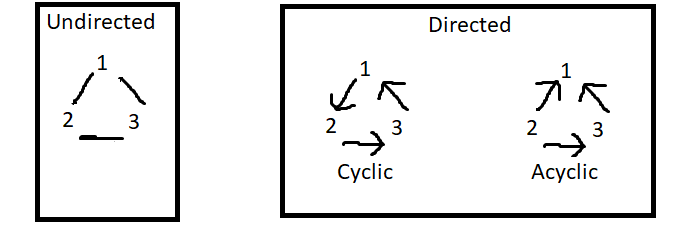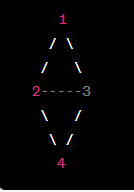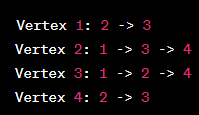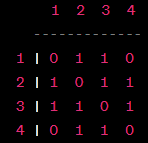×

Search anything:

# Implement graph in Ruby [adjacency list and matrix]

#### Ruby ProgrammingGet this book -> Problems on Array: For Interviews and Competitive Programming

1. Introduction
2. What is a graph
5. Conclusion

## Introduction

In this article at OpenGenus, we are going to be talking about the graph data structure and how it is used with the programming language of Ruby.

## What is a graph

A graph is a data structure used to show data with objects and the relationship between those objects. Objects on a graph are also refered to as vertices or nodes and the connection between those vertices(nodes) are known as edges. A graph can either be undirected or directedIn the example shown above, the numbers 1,2,3 would be the nodes and the lines connecting them would be the edges. Undirected graphs dont have any specific or set flow (direction) to follow we just have nodes that are connected to each other but directed graphs are connected in specific directions when the connection can complete a cycle going in one direction (as shown in the example Cyclic) 1 flows to 2 then 2 flows to 3 and 3 flows back to 1 making a complete cycle,this is a DCG(directed cyclic graph), on the other hand the DAG(directed acyclic graph) is not flowing in one direction and so does not complete a cycle.

Ok let's get to it. How would we write the code for a Graph using ruby? Well they are to ways to represent this Adjacency list and Adjacency matrix, first we will see the undirected adjacency list method. With adjacency list each node is represented as a key in a hash table and its value is an array of nodes that it is connected to.

``````class Graph
def initialize
@nodes = {}
end

@nodes[node] = []
end

@nodes[node1] << node2
@nodes[node2] << node1
end

def neighbors(node)
@nodes[node]
end
end
``````

With this Graph class, you can create a new graph, add a node, add an edge and get a list of the nodes neighbors

``````graph = Graph.new
graph.neighbors("B")
# => ["A", "C"]
``````

So we created a new graph, added 3 nodes A,B,C and then added the edges/connections between A and B , B and C, we then asked for the neighbors of B using the neighbors method and we get our answer A, C.

Now let us code the undirected adjacency matrix.

``````class Graph
def initialize(num_nodes)
@num_nodes = num_nodes
@adj_matrix = Array.new(num_nodes) { Array.new(num_nodes, 0) }
end

end

def has_edge?(node1, node2)
end
end
``````

Here things are a bit different, we have a two-dimensional array where each element (i, j) represents whether there is an edge between node i and node j.

``````graph = Graph.new(3)
``````

We create a new graph by specifying the number of nodes we want, then we add the edges(connections). We can then ask if a connection is present between the nodes

``````graph.has_edge?(0, 1)
# => true
``````

## Conclusion

To recap we found out what a graph is and how it is used in ruby, I will conclude by stating the difference between adjacency list and adjacency matrix.1. Space complexity: adjacency list is more space-efficient for sparse graphs, while adjacency matrix is more space-efficient for dense graphs.
2. Traversal efficiency: adjacency list is more efficient for sparse graphs, while adjacency matrix is more efficient for dense graphs.
3. Edge existence check efficiency: adjacency matrix is more efficient for checking whether there is an edge between two vertices.